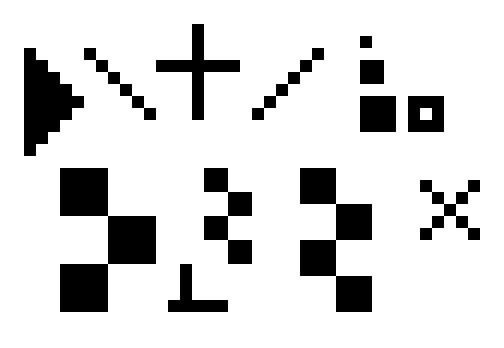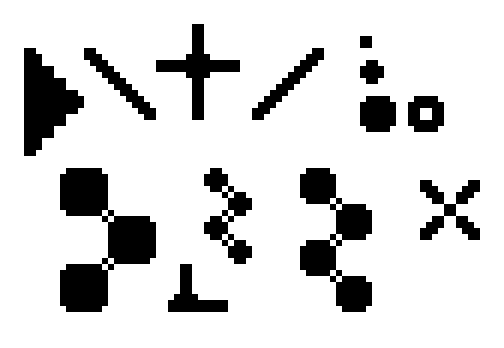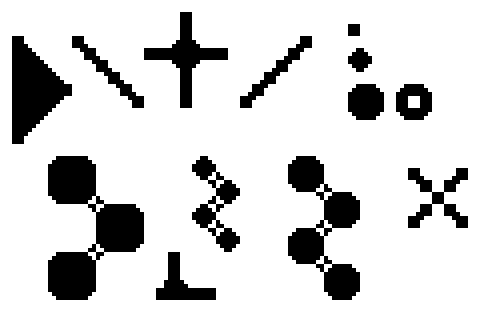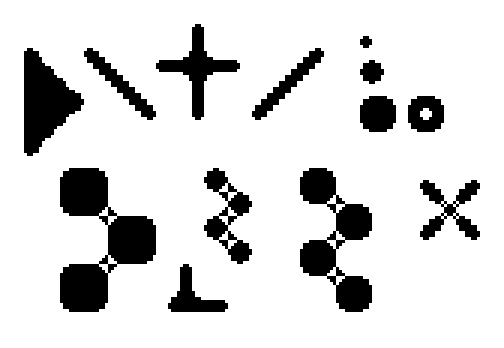## Scale2x Algorithm

The effect works repeating a computation pattern for every pixel of the original image. The pattern starts from a square of 9 pixels and expands the central pixel computing 4 new pixels.

Starting from this pattern :

 A B C D E F G H I

The central pixel E is expanded in 4 new pixels:

 E0 E1 E2 E3

with these rules (in C language) :

```E0 = D == B && B != F && D != H ? D : E;
E1 = B == F && B != D && F != H ? F : E;
E2 = D == H && D != B && H != F ? D : E;
E3 = H == F && D != H && B != F ? F : E;
```

which can be rewritten as :

```E0 = D == B && B != H && D != F ? D : E;
E1 = B == F && B != H && D != F ? F : E;
E2 = D == H && B != H && D != F ? D : E;
E3 = H == F && B != H && D != F ? F : E;
```

and optimized as:

```if (B != H && D != F) {
E0 = D == B ? D : E;
E1 = B == F ? F : E;
E2 = D == H ? D : E;
E3 = H == F ? F : E;
} else {
E0 = E;
E1 = E;
E2 = E;
E3 = E;
}
```

The image border is computed reusing the value of the nearest pixel on the border when the value of a pixel over the border is required.

## Scale3x Algorithm

Starting from this pattern :

 A B C D E F G H I

The central pixel E is expanded in 9 new pixels:

 E0 E1 E2 E3 E4 E5 E6 E7 E8

with these rules (in C language) :

```E0 = D == B && B != F && D != H ? D : E;
E1 = (D == B && B != F && D != H && E != C) || (B == F && B != D && F != H && E != A) ? B : E;
E2 = B == F && B != D && F != H ? F : E;
E3 = (D == B && B != F && D != H && E != G) || (D == H && D != B && H != F && E != A) ? D : E;
E4 = E
E5 = (B == F && B != D && F != H && E != I) || (H == F && D != H && B != F && E != C) ? F : E;
E6 = D == H && D != B && H != F ? D : E;
E7 = (D == H && D != B && H != F && E != I) || (H == F && D != H && B != F && E != G) ? H : E;
E8 = H == F && D != H && B != F ? F : E;
```

and optimized as:

```if (B != H && D != F) {
E0 = D == B ? D : E;
E1 = (D == B && E != C) || (B == F && E != A) ? B : E;
E2 = B == F ? F : E;
E3 = (D == B && E != G) || (D == H && E != A) ? D : E;
E4 = E;
E5 = (B == F && E != I) || (H == F && E != C) ? F : E;
E6 = D == H ? D : E;
E7 = (D == H && E != I) || (H == F && E != G) ? H : E;
E8 = H == F ? F : E;
} else {
E0 = E;
E1 = E;
E2 = E;
E3 = E;
E4 = E;
E5 = E;
E6 = E;
E7 = E;
E8 = E;
}
```

## Scale4x Algorithm

The Scale4x effect is simply the Scale2x effect applied two times.

## Examples

The following are some examples that show the application of the effects.originalscale2xscale3xscale4x